## 数字信号处理第3部分—傅里叶变换## 硬件

• • Arduino Uno
• • 用于Arduino的MAX9812L驻极体麦克风传感器板

• Arduino IDE
• MATLAB

## 步骤 1：傅里叶变换简介

FT也会在图像和视频压缩中用到。例如，jpgmp3是使用了快速傅里叶变换（FFT）算法的图像和声音的数字格式。由于必须使用模数转换器将每个连续的模拟信号转换为数字信号，所以需要以特定频率对这些信号进行采样。这样，我们可以利用离散傅里叶变换获取离散信号。f1 = 1;
f2 = 0.5;
f3 = 1.5;
f4 = 4;
t = 0:0.01:4;
A1 = 0.5;
A2 = 2.5;
A3 = 7.5;
A4 = 3.5;

x1 = A1*sin(2*pi*f1*t)
x2 = A2*sin(2*pi*f2*t)
x3 = A3*sin(2*pi*f3*t)
x4 = A4*sin(2*pi*f4*t)

x = x1 + x2+ x3+ x4

figure %Figure 2
subplot(2,1,1)
plot(t,x,t,x1)
subplot(2,1,2)
plot(t,x1)figure %Figure 3
subplot(3,1,1)
plot(t,x,t,x1+x2)
subplot(3,1,2)
plot(t,x2)
subplot(3,1,3)
plot(t,x1+x2)

1. 1.subplot(3,1,1)：在同一坐标图中绘制两个图形，蓝色表示初始信号，由不同频率的正弦波叠加而成，绿色表示获得原始信号之前的所有步骤中所添加信号的总和。
2. 2.subplot(3,1,2)：当前步骤中所添加的信号，由另一个幅度和频率来表达。
3. 3.subplot(3,1,3)：信号总和 – subplot(3,1,1) 中的绿色信号，没有重叠部分。figure %Figure 4
subplot(3,1,1)
plot(t,x,t,x1+x2+x3)
subplot(3,1,2)
plot(t,x3)
subplot(3,1,3)
plot(t,x1+x2+x3)figure %Figure 5
subplot(3,1,1)
plot(t,x,t,x1+x2+x3+x4)
subplot(3,1,2)
plot(t,x4)
subplot(3,1,3)
plot(t,x1+x2+x3+x4)## 步骤2：背后的算法• • 时域中的点数等于频域中的点数。
• • 假设您有一个包含DC值的正弦波 – 频谱中的第一个点将具有零频率值（DC值），而下一个点为正弦频率。
• • 当从时域转为频域时，可以使用第n个点的频率进行绘制：f = (n-1) SR/N，其中N为样本点数。
• • 频率为f = 1/T，其中T为周期。

t = 0:1/1000:1-1/1000;
x = sin(2*pi*30*t);
plot(t,x)y = fft(x);
f = (0:length(y)-1)*1000/length(y);
plot(f,abs(y))>> length(t)

ans =

1000##### Tiberia Todeila

Tiberia目前是罗马尼亚布加勒斯特理工大学电气工程学院的大四学生。 她非常热衷于设计和开发让日常生活更轻松的智能家居设备。

### 相关文章

1.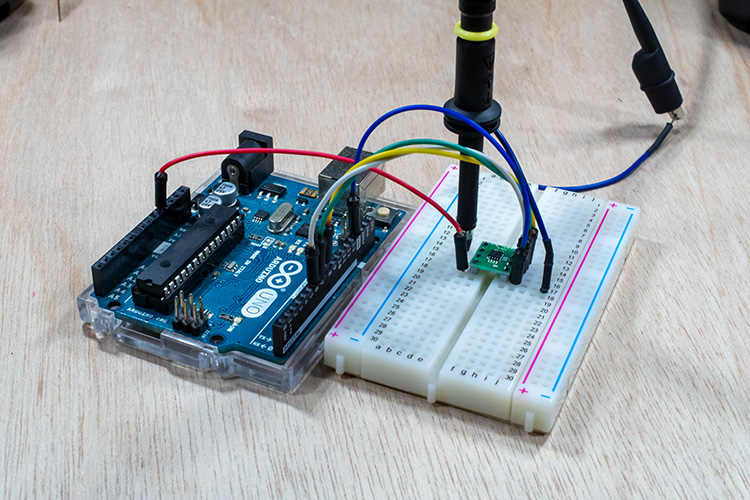#### 使用D/A转换器灵活控制电压并使用Arduino输出模拟信号的方法

2.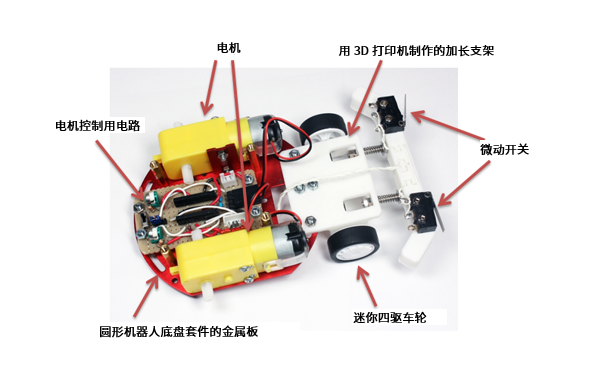#### 在最后一刻停下来！用Arduino和距离传感器制作小鸡赛车！（第3篇•最终篇）

3.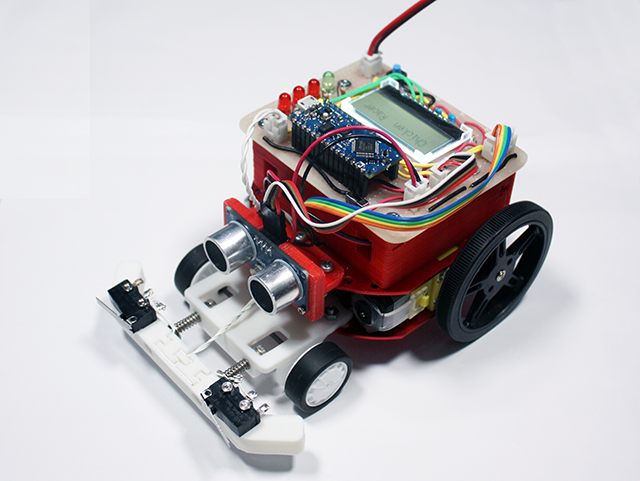#### 在最后一刻停下来！用Arduino和距离传感器制作小鸡赛车！（第2篇）

4.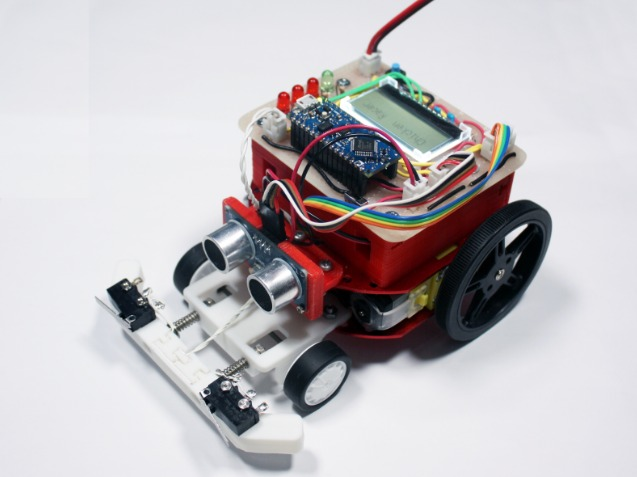#### 在最后一刻停下来！用Arduino和距离传感器制作小鸡赛车！（第1篇）

5.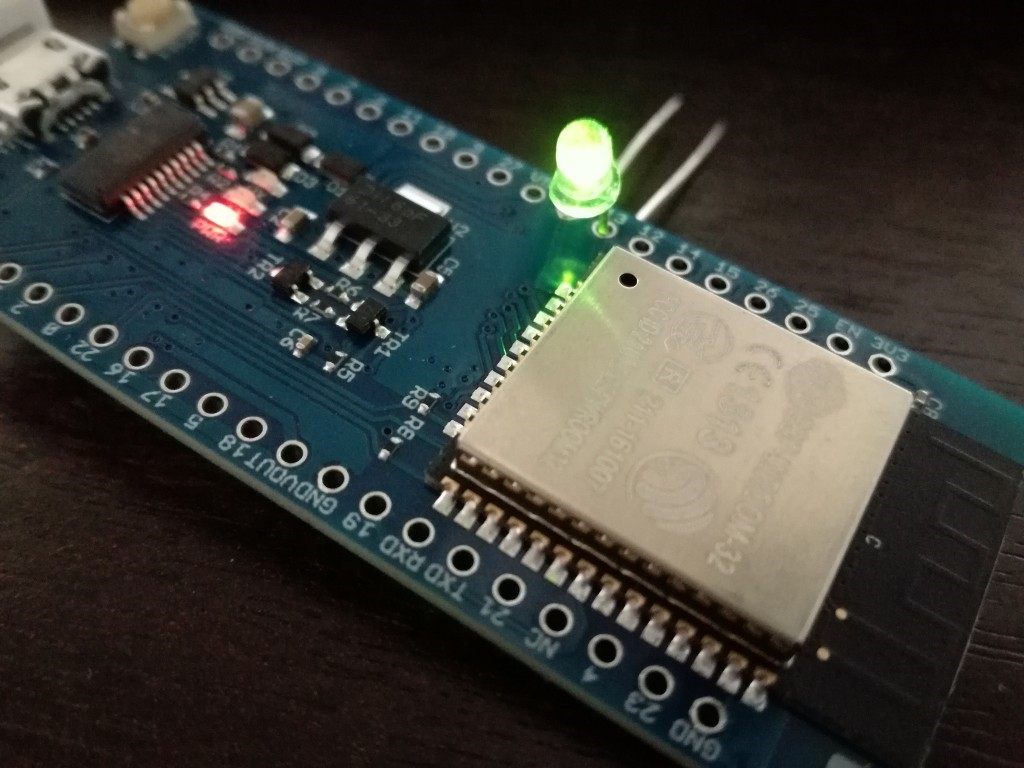#### 通过Arduino使用ESP-WROOM-32（环境搭建和配置-LED闪烁篇）

6.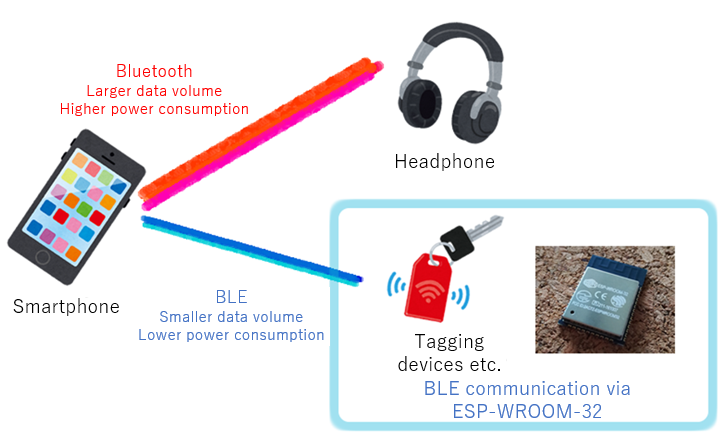#### 通过Arduino使用ESP-WROOM-32（Bluetooth-BLE连接篇）

7.#### 六种可以用于扩展物联网应用的DIY项目

8.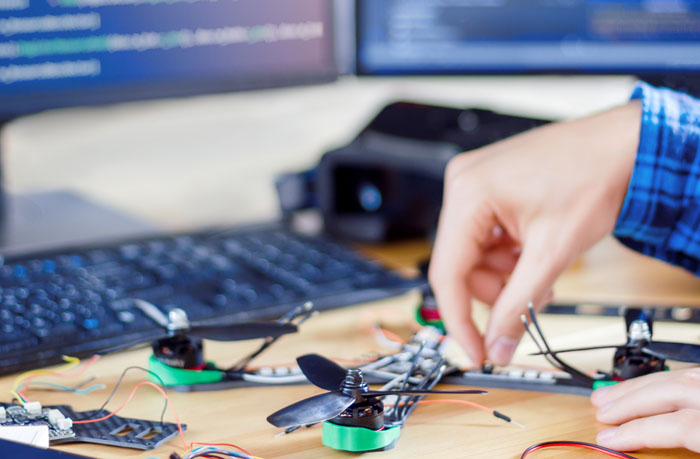#### 使用Arduino的五大DIY无人机项目

9.